## NCERT Solutions for Class 10 Science Chapter 13 Magnetic Effects of Electric Current

These Solutions are part of NCERT Solutions for Class 10 Science. Here we have given NCERT Solutions for Class 10 Science Chapter 13 Magnetic Effects of Electric Current. LearnInsta.com  provides you the Free PDF download of NCERT Solutions for Class 10 Science (Physics) Chapter 13 – Magnetic Effects of Electric Current solved by Expert Teachers as per NCERT (CBSE) Book guidelines. All Chapter 13 – Magnetic Effects of Electric Current Exercise Questions with Solutions to help you to revise complete Syllabus and Score More marks.

NCERT Questions

In Text Questions

Question 1.
Why does a compass needle get deflected when brought near a bar magnet ?
Compass needle is a small magnet which experiences a force in the magnetic field of a bar magnet. Due to this force, it gets deflected.

More Resources

Question 2.
Draw magnetic field lines around a bar magnet. (CBSE 2011, 2012, 2013, 2014)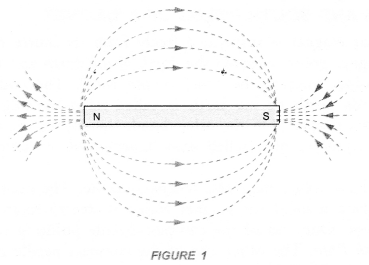Question 3.
List the properties of magnetic field lines. (CBSE 2015)
Or
Write four properties of magnetic field lines. (CBSE (Delhi) 2010, 2011, 2012, 2013)

1. Magnetic field lines are closed continuous curves.
2. The tangent at any point on the magnetic field line gives the direction of the magnetic field at that point.
3. No two magnetic field lines can cross each other.
4. Magnetic field lines are crowded in a region of strong magnetic field and magnetic field lines diverge in a region of weak magnetic field.

Question 4.
Why do not two magnetic field lines intersect each other ? (CBSE 2011, 2012, 2015)
Or
No two magnetic field lines can intersect each other. Explain. (CBSE 2010, 2014)
The tangent at any point on a magnetic field line gives the direction of magnetic field at that point. If two magnetic field lines cross each other, then at the point of intersection, there will be two tangents. Hence, there will be two directions of the magnetic field at the point of intersection. This is not possible. Hence, no two magnetic field lines can cross each other.Question 5.
Consider a circular loop of wire lying in the plane of the table. Let the current pass through the loop clockwise. Apply the right hand rule to find out the direction of magnetic field inside and outside the loop.
(CBSE (Delhi) 2009)
Magnetic field inside the loop is perpendicular to the plane of table and in the downward direction. However, outside the loop, magnetic field is perpendicular to the plane of the table and in the upward direction.

Question 6.
The magnetic field in a given region is uniform. Draw a diagram to represent it.
(CBSE 2011, 2012, 2015)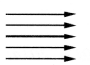Question 7.
Choose the correct option :
The magnetic field inside a long straight solenoid carrying current is :
(a) zero
(b) decreases as we move towards its ends .
(c) increases as we move towards its ends
(d) is the same at all points.
(b).

Question 8.
Which of the property a proton can change when it moves freely in a magnetic field ? (There may be more than one correct answer).
(a) mass
(b) speed
(c) velocity
(d) momentum.
A force acts on a proton when it moves freely in a magnetic field. Hence its velocity and momentum can change.

Question 9.
In activity 13-7 (NCERT book), how do we think the displacement of rod AB will be affected if

1. current in rod AB is increased
2. a stronger horse shoe magnet is inserted
3. length of the rod AB is increased.

Force acting on a current carrying conductor of length l placed perpendicular to magnetic field B is given by F = B I l

1. When I increase, F also increases. Hence the displacement of the rod increases.
2. When a stronger horse shoe magnet is inserted, magnetic field at B increases. So force also increases. Hence displacement increases.
3. When l increases, force increases and hence displacement increases.

Question 10.
A positively charged partical (alpha partical) projected towards west is deflected towards north by a magnetic field. The direction of magnetic field is
(a) towards south
(b) towards east
(c) downward
(d) upward.
(d).

Question 11.
State Fleming’s left hand rule with a labelled diagram. (CBSE 2005, 2010, 2011, 2012)
Fleming’s left hand rule
Statement : Stretch the left hand such that the thumb, first finger and the central finger are mutually perpendicular to each other. If the First finger points in the direction of the magnetic Field and the Central finger points in the direction of Current, then the thumb will point in the direction of Motion (or Force) as shown in figure 24.Thus, in Fleming’s left hand rule, first finger shows the direction of the magnetic field. The central finger shows the direction of electric current flowing in the conductor. Thumb shows the direction of force on the conductor or the direction of motion of the conductor

Question 12.
What is the principle of electric motor ? (CBSE 2012)
Electric motor works on the principle that a current carrying conductor placed perpendicular to a magnetic field experiences a force.

Question 13.
What is the role of the split ring in an electric motor ?
The split-ring in an electric motor reverses the direction of current in the armature coil of the motor. Therefore, the direction of the force acting on the two arms of the coil is also reversed. As a result of this, the coil of d.c. motor continues to rotate in the same direction.

Question 14.
Explain different ways to induce current in a coil. (CBSE 2010, 2011, 2012)

1. By moving a bar magnet towards or away from the coil.
2. By placing a closed coil near another coil connected across a battery.

Question 15.
State the principle of electric generator.
It is based on the principle of electromagnetic induction. That is, the changing magnetic field induces current in the coil.

Question 16.
Name some sources of direct current. (Bihar Board 2012, 2015)
A dry cell, a battery, a solar cell, d.c. generator etc. are some sources of direct current.

Question 17.
Which source produces alternating current ?
AC generator (which converts mechanical energy into alternating current or electricity) and an oscillator (a device which converts D.C. into A.C.) are the sources which produce alternating current.

Question 18.
Choose the correct option :
A rectangular coil of copper wires is rotated in a magnetic field. The direction of the induced current changes once in each
(a) two revolutions
(b) one revolution
(c) half revolution
(d) one-fourth revolution,
(c).

Question 19.
Name two safety measures commonly used in electric circuit and appliances.
(CBSE 2010, 2012, 2014, 2015)

1. Electric fuse and
2. earthing.

Question 20.
An electric oven of 2 kW power rating is operated in a domestic electric circuit (220 V) that has a current rating of 5 A. What result do you expect ? Explain. (CBSE 2010, 2011, 2012, 2013, 2014)
P = 2 kW = 2000 W and V = 220 VThis shows that current flowing through the oven is more than the current rating (5 A). Hence, the fuse in the circuit melts and oven is saved from damage.

Question 21.
What precautions should be taken to avoid the overloading of domestic electric circuit ? (CBSE 2010, 2011, 2012, 2015)
Or
Write any two precautions to be taken to avoid overloading of a domestic electric circuit.

1.  We should not connect many appliances in the same socket.
2. Electrical appliances of high power rating should not be switched on simultaneously.

NCERT Chapter End Exercises

Question 1.
Which of the following correctly describes the magnetic field near a long straight wire ?
(a) the field consists of straight lines perpendicular to the wire
(b) the field consists of straight lines parallel to the wire
(c) the field consists of radial lines originating from the wire
(d) the field consists of concentric circles centered on the wire.
(d).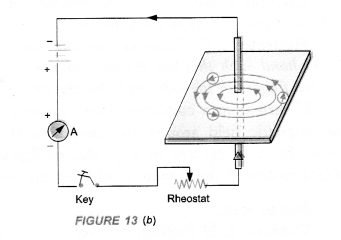Question 2.
The phenomena of electromagnetic induction is
(a) the process of charging a body
(b) the process of generating magnetic field due to current passing through a coil
(c) producing induced current in a coil due to relative motion between a magnet and the coil
(d) the process of rotating a coil of an electric motor.
(c).

Question 3.
The device used for producing electric current is called a
(a) generator
(b) galvanometer
(c) ammeter
(d) motor. (Bihar Board 2012)
(a).

Question 4.
The essential difference between an AC generator and a DC generator is that
(a) AC generator has an electromagnet while a DC generator has permanent magnet
(b) DC generator will generate a higher voltage
(c) AC generator will generate & higher voltage
(d) AC generator has slip rings while the DC generator has a commutator.
(d).

Question 5.
At the time of short circuit, the current in the ciruit
(a) reduces substantially
(b) does not change
(c) increases heavily
(d) vary continuously. (Bihar Board 2012)
(c).

Question 6.
State whether the following statements are true or false :
(a) an electric motor converts mechanical energy into electrical energy ‘
(b) an electric generator works on the principle of electromagnetic induction
(c) the field at the centre of a long circular coil carrying current will be parallel straight lines
(d) a wire with a green insulation is usually the live wire.
(a) False. It converts electrical energy into mechanical energy.
(b) True.
(c) True.
(d) False. Five wire has red insulation cover.

Question 7.
List three sources of magnetic fields. (CBSE 2013)

1. a permanent magnet
2. a current carrying conductor
3. a current carrying solenoid.

Question 8.
How does a solenoid behave like a magnet ? Can you determine the north and south poles of a current carrying solenoid with the help of a bar magnet. Explain. (CBSE 2012, 2013)
When electric current flows through a solenoid, magnetic field is set up around the solenoid. The pattern of the magnetic field is same as that of the magnetic field of a bar magnet. One end of the solenoid behaves as north pole and the other end of the solenoid behaves as south pole.
To determine the north and south poles of a current carrying solenoid with the help of a bar magnet, suspend it with a strong thread. Now bring the north pole of a bar magnet towards one end of the solenoid. If the solenoid attracts towards the magnet, then that face of the solenoid is south pole. If the bar magnet moves away from the solenoid, then that face of the solenoid is the north pole.

Question 9.
When is the force experienced by a current carrying conductor placed in magnetic field is the largest ?
[CBSE, (All India) 2009, 2010]
Or
Under what condition does a current carrying conductor kept in a magnetic field experience maximum force ? (CBSE 2011, 2012, 2014, 2015)
When current carrying conductor is placed perpendicular to the magnetic field.

Question 10.
Think you are sitting in a chamber with your back to one wall. An electron beam moving horizontally from back wall towards the front wall, is deflected by a strong magnetic field to your right side. What is the direction of magnetic field ?
Movement from electron beam from back wall to the front wall is equivalent to the flow of electric current from front wall to the back wall. The deflection of the beam means, the force is acting towards our right side. According to Fleming’s Left Hand Rule, the direction of magnetic field is vertically downward. That is, the magnetic field is perpendicular to the plane of the paper and directed inward.
Such magnetic field is shown by ⊗.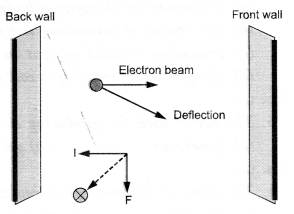Question 11.
Draw a labelled diagram of an electric motor. Explain its principle and working. What is the function of a split ring in an electric motor ?
Electric motor converts electrical energy into mechanical energy.
Principle: Electric Motor is based on the fact that a current carrying conductor placed perpendicular to the magnetic field experiences a force.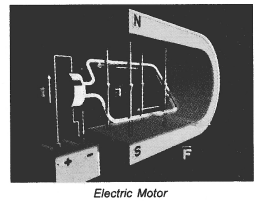1. Armature coil: It consists of a single loop of an insulated copper wife in the form of a rectangle. Rectangle ABCD shown in figure 25 is an armature coil.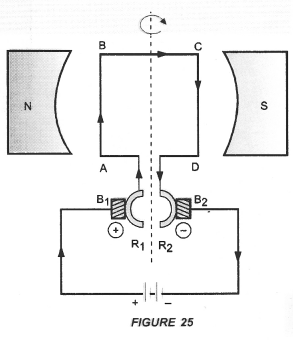2. Strong field magnet: Armature coil is placed between two pole pieces (N and S poles) of a strong magnet. This magnet provides a strong magnetic field.
3. Split-ring type Commutator. It consists of two halves (R1 and R2) of a metallic ring. The two ends of the armature coil are connected to these two halves of the ring. Commutator reverses the direction of current in the armature coil.
4. Two carbon brushes B1 and B2 press against the commutator. These brushes act as the contacts between the commutator and the terminals of the battery.
5. A battery is connected across the carbon brushes. This battery supplies the current to the armature coil.

Question 12.
Name some devices in which electric motors are used.
Electric cars, rolling mills, electric fans, hair dryers, mixers, blenders etc.

Question 13.
A coil of insulated copper wire is connected to a galvanometer. What will happen if a bar magnet is

1. pushed into the coil,
2. withdrawn from inside the coil,
3. held stationary inside the coil ?

Name the phenomenon involved in the above cases.
(CBSE 2010, Term I, 2011, 2012, 2013, 2014)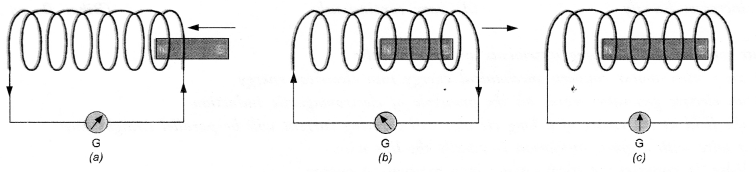1. When a bar magnet is pushed into the coil, induced current flows through the coil due to the phenomenon of electromagnetic induction. This induced current is indicated by the deflection of the needle of the galvanometer as shown in figure (a).
2. When a bar magnet is withdrawn from inside the coil, again induced current flows through the coil due to the phenomenon of electromagnetic induction. In this case, the direction of induced current is opposite to the direction of the current in case (i) as shown in figure (b).
3. When the bar magnet is held stationary inside the coil, there is no change in magnetic field around the coil. Hence, no induced current flows through the coil. Therefore, galvanometer shows no deflection as shown in figure (c).
Phenomenon involved in electromagnetic induction.

Question 14.
Two circular coils A and B are placed close to each other. If the current in the coil A is changed, will some current be induced in the coil B 1 Give reason. (CBSE 2010, 2012)
When current in coil A is changed, a changing magnetic field is set up around it. This changing magnetic field also links with coil B and hence some current will be induced in coil B due to electromagnetic induction.

Question 15.
State the rule to determine the direction of a

1. magnetic field produced around a straight conductor carrying current,
2. force experienced by a current-carrying straight conductor placed in a magnetic field which is perpendicular to it, and
3. current induced in a coil due to its rotation in a magnetic field.
(CBSE 2010, 2011, 2014)

1. Right hand thumb rule,
2. Fleming’s left hand rule,
3. Fleming’s right hand rule.

Question 16.
Explain the underlying principle and working of an electric generator by drawing a labelled diagram. What is the function of brushes ?
An electric device used to convert mechanical energy (kinetic energy) into electrical energy (electricity) is called an electric generator.
Principle: Electric generator works on the principle of electromagnetic induction. When the coil of electric generator rotates in a magnetic field, induced current flows in the circuit connected with the coil.
types of electric generator

1. AC generator
2. DC generator

AC generator: AC generator converts mechanical energy into electrical energy in the form of alternating current or AC.
DC generator: DC generator converts mechanical energy into electrical energy in the form of direct current or DC.                                                AC Generator Construction : The main components of AC generator are (Figure 33) :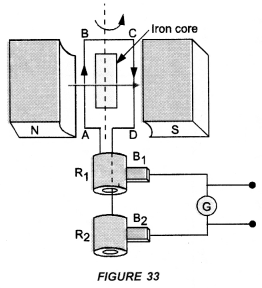1. Armature : Armature coil (ABCD) consists of large number of turns of insulated copper wire wound over a soft iron core.
2. Strong field magnet : A strong permanent magnet or an electromagnet whose poles (N and S) are cylindrical in shape is a field magnet. The armature coil rotates between the pole pieces of the field magnet. The uniform magnetic field provided by the field magnet is perpendicular to the axis of rotation of the coil.
3. Slip Rings : The two ends of the armature coil are connected to two brass slip rings R1 and R2. These rings rotate along with the armature coil. Rings R1 and R2 are at different heights.
4. Brushes : Two carbon brushes (B1 and B2), are pressed against the slip rings. The brushes are fixed while slip rings rotate along with the” armature. These brushes are connected to the external circuit across which the output is obtained.

Working : When the armature coil ABCD rotates in the magnetic field provided by the strong field magnet, it cuts the magnetic field lines. Thus, the changing magnetic field produces induced current in the coil. The direction  of the induced current in the coil is determined by the Fleming’s right hand rule.
The current flows out through the brush B1 in one direction in the first half of the revolution and through the brush B2 in the next half revolution in the reverse direction. This process is repeated. Therefore, induced current produced is of alternating nature. Such a current is called alternating current.
DC generator or Dynamo Construction:

1. Armature coil. It consists of large number of turns of insulated copper wire wound on iron core in the form of a rectangle coil. Rectangle coil ABCD shown in figure 34 is an armature coil.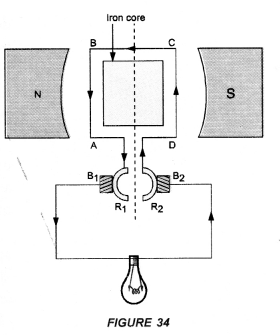2. Strong field magnet. Armature coil is placed between two pole pieces (N and S poles) of a strong magnet. This magnet provides a strong magnetic field.
3. Split-ring Type Commutator. It consists of two halves (R1 and R2) of a metallic ring. The two ends of the armature coil are connected to these two halves of the ring.
4. Two carbon brushes B1 and B2 press against the commutator.
5. The output is shown by the glowing bulb connected across the carbon brushes.

Working of d.c. generator: When the coil of d.c. generator rotates in the magnetic field, induced potential difference is produced in the coil. This induced potential difference gives rise to the flow of current through the bulb and hence the bulb glows.
In d.c. generator, the flow of current in the circuit is in the same direction as long as the coil rotates in the magnetic field. This is because one brush is always in contact with the arm of the armature moving up and the other brush is in contact with the arm of the armature moving downward in the magnetic field.
Note: AC generator can be converted into DC generator by replacing slip rings used in AC generator by a split ring type commutator.

Question 17.
When does an electric short circuit occur ? (CBSE 2013)